NCERT Questions

Class 10
Chapter 12 Class 10 - Electricity

## A copper wire has a diameter 0.5 mm and resistivity of 1.6 × 10–8 Ω m. What will be the length of this wire to make its resistance 10 Ω? How much does the resistance change if the diameter is doubled?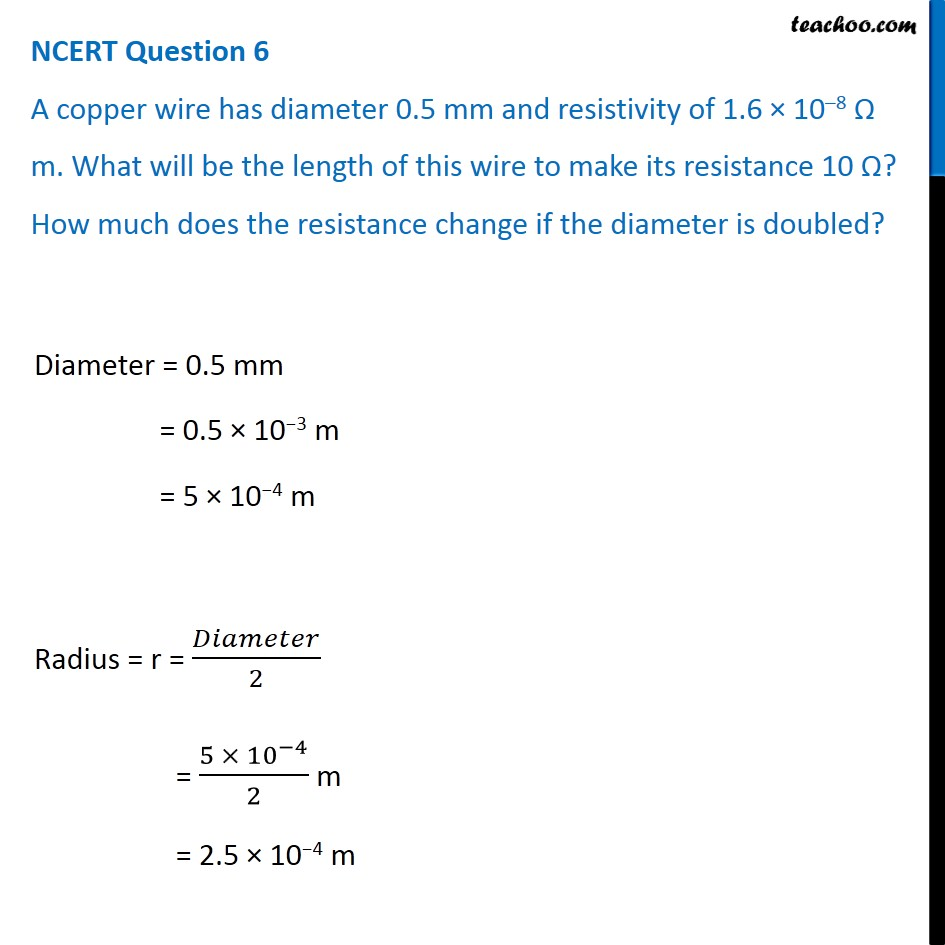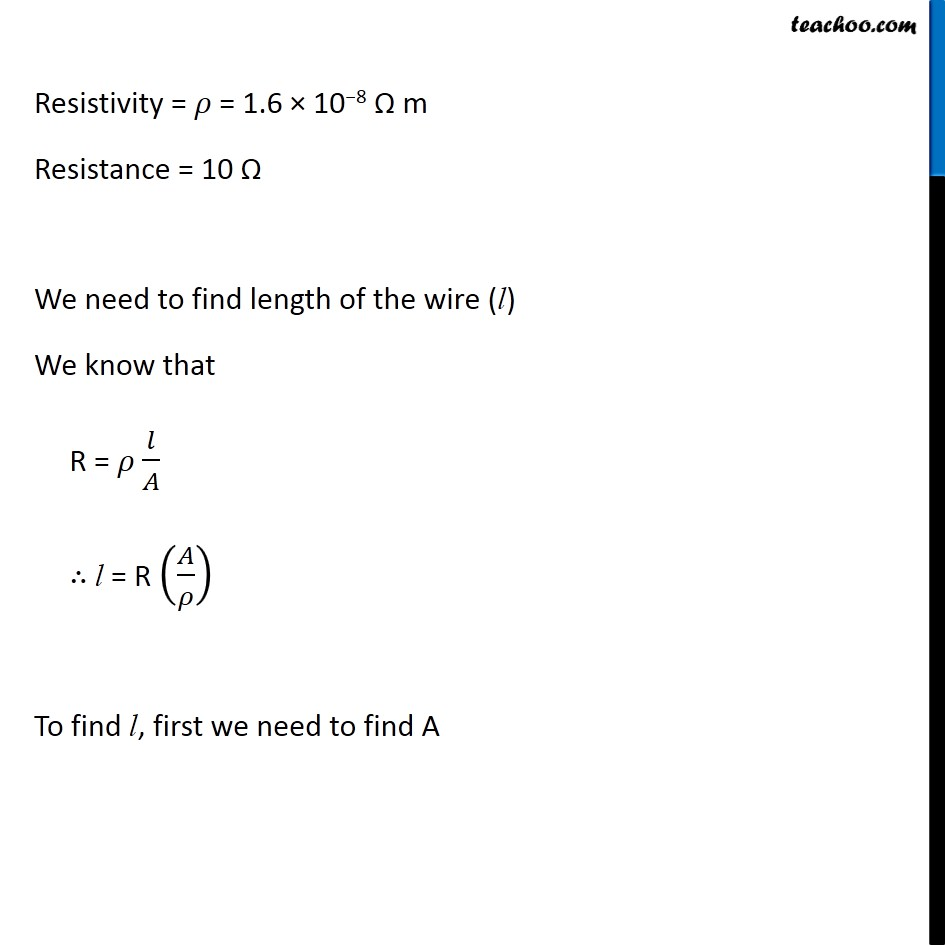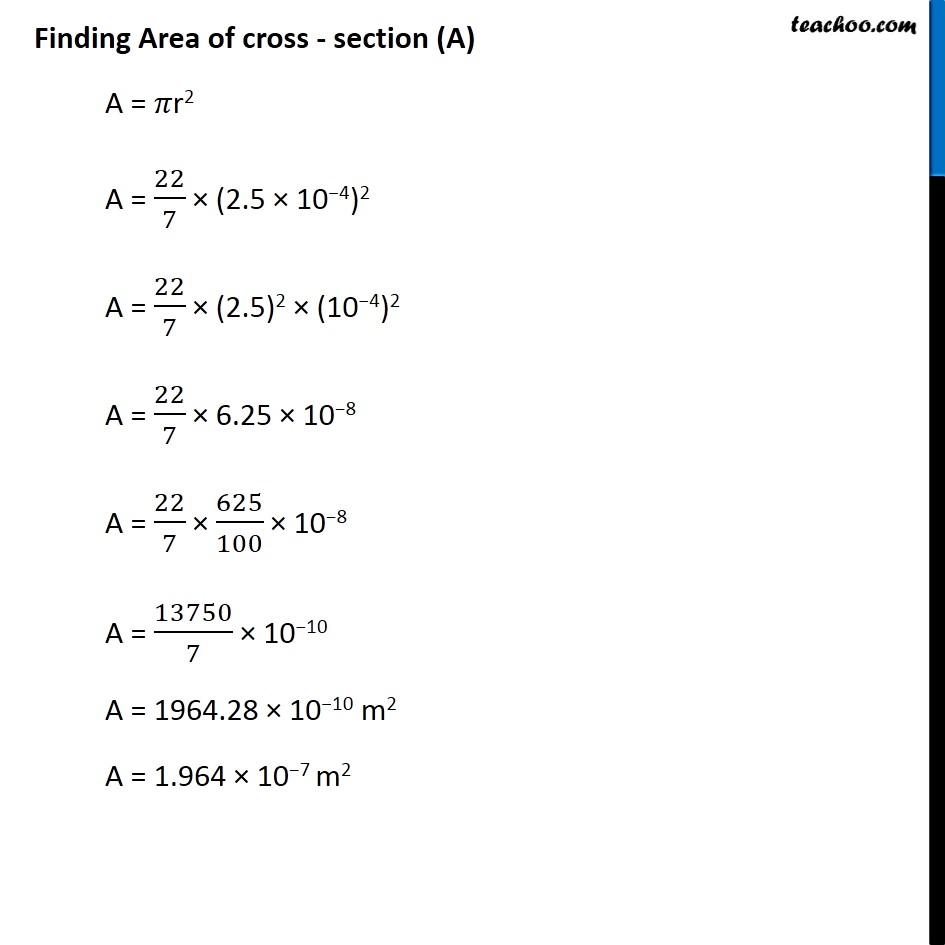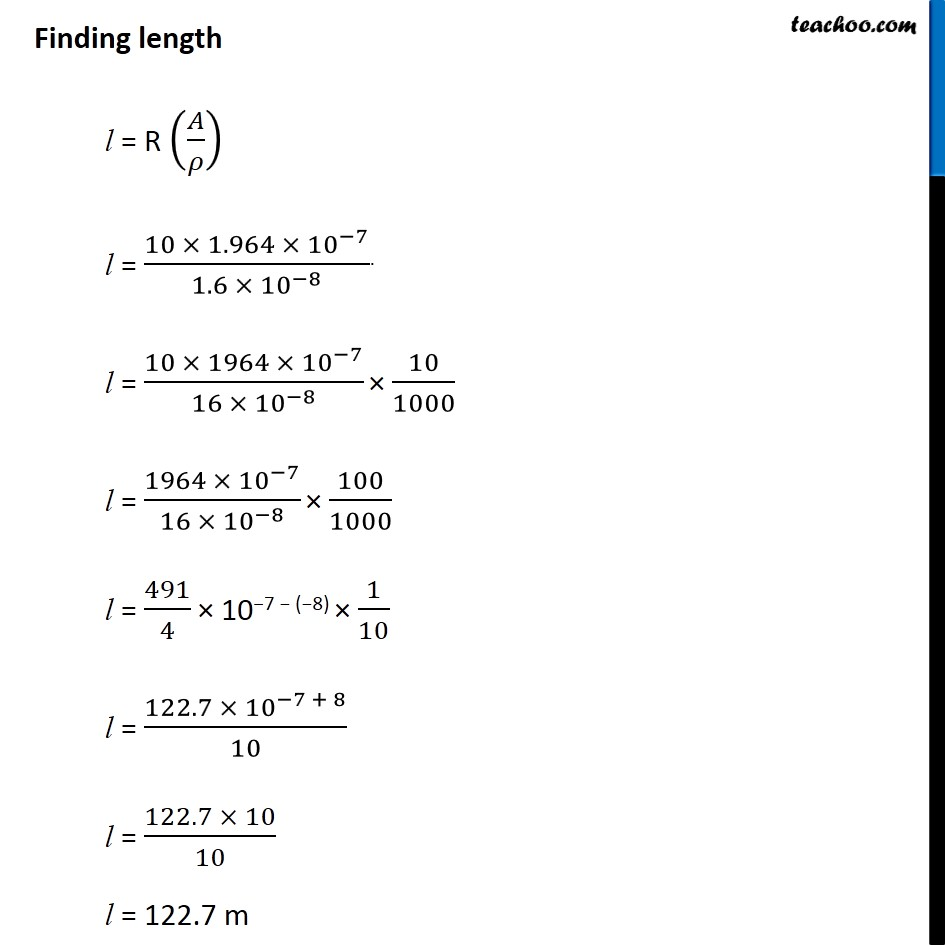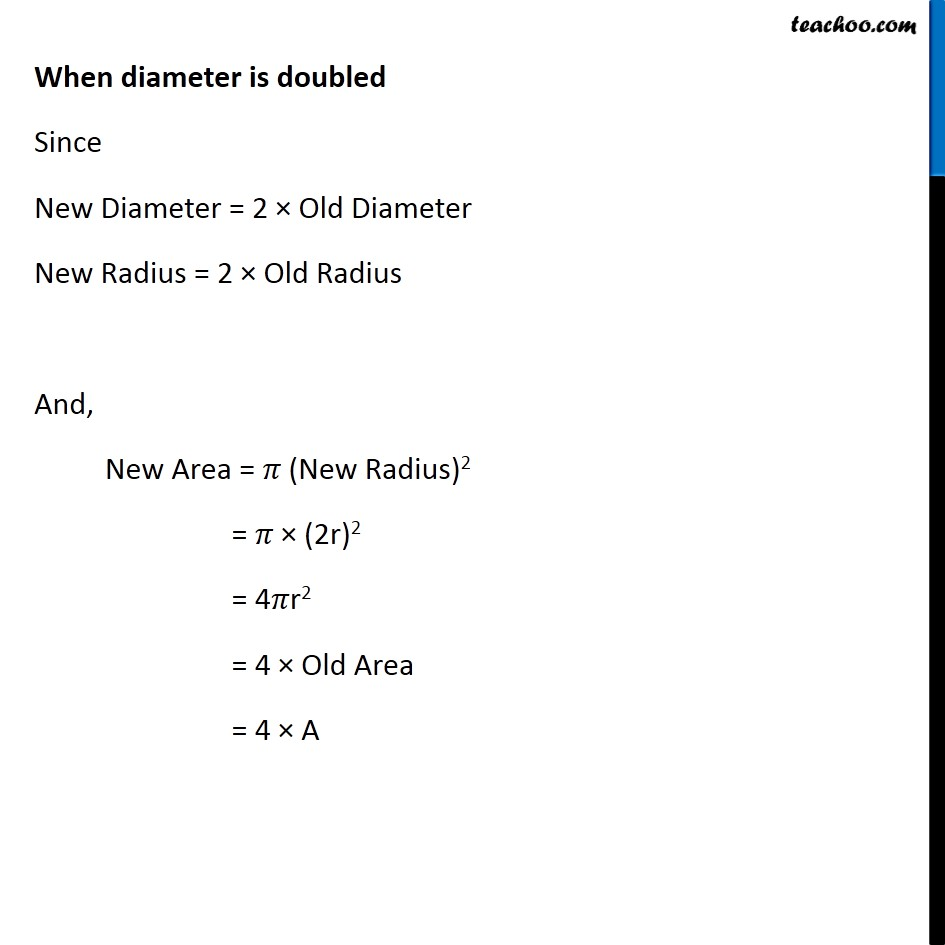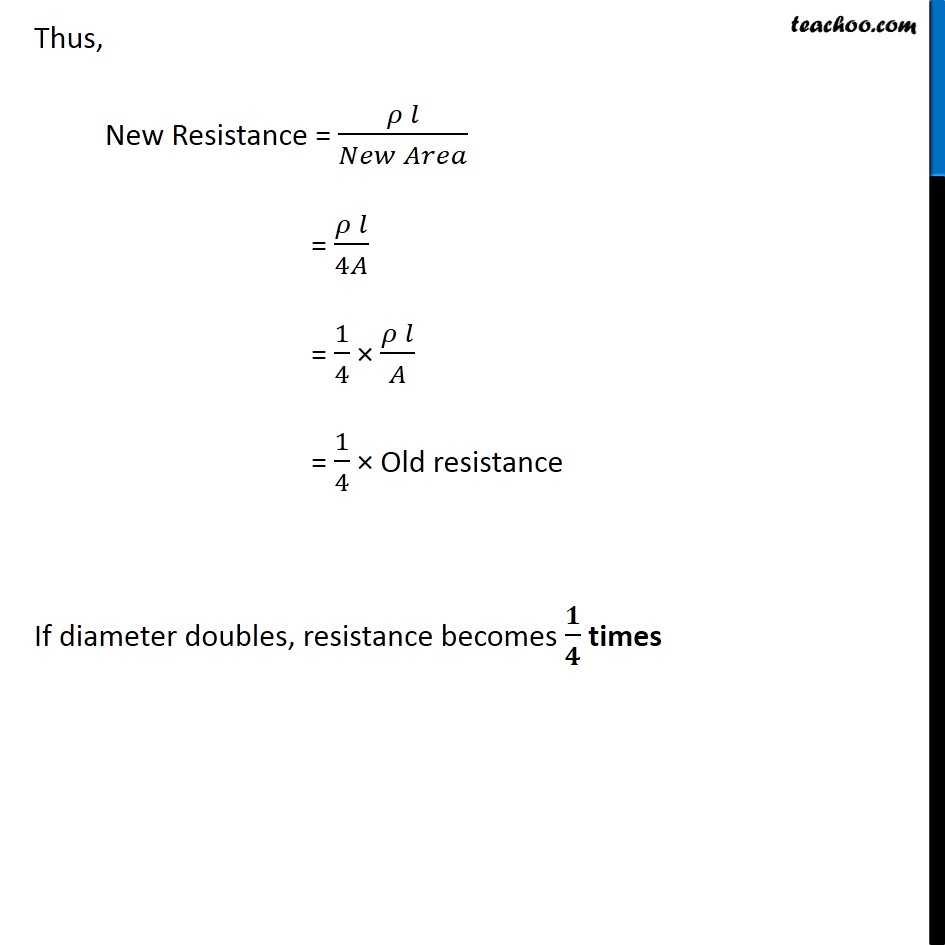Learn in your speed, with individual attention - Teachoo Maths 1-on-1 Class

### Transcript

NCERT Question 6 A copper wire has diameter 0.5 mm and resistivity of 1.6 × 10–8 Ω m. What will be the length of this wire to make its resistance 10 Ω? How much does the resistance change if the diameter is doubled? Diameter = 0.5 mm = 0.5 × 10−3 m = 5 × 10−4 m Radius = r = 𝐷𝑖𝑎𝑚𝑒𝑡𝑒𝑟/2 = (5 × 〖10〗^(−4))/2 m = 2.5 × 10−4 m Resistivity = 𝜌 = 1.6 × 10−8 Ω m Resistance = 10 Ω We need to find length of the wire (l) We know that R = 𝜌 𝑙/𝐴 ∴ l = R (𝐴/𝜌) To find l, first we need to find A Finding Area of cross - section (A) A = 𝜋r2 A = 22/7 × (2.5 × 10−4)2 A = 22/7 × (2.5)2 × (10−4)2 A = 22/7 × 6.25 × 10−8 A = 22/7 × 625/100 × 10−8 A = 13750/7 × 10−10 A = 1964.28 × 10−10 m2 A = 1.964 × 10−7 m2 Finding length l = R (𝐴/𝜌) l = (10 × 1.964 × 〖10〗^(−7))/(1.6 × 〖10〗^(−8) ). l = (10 × 1964 × 〖10〗^(−7))/(16 × 〖10〗^(−8) ) × 10/1000 l = (1964 × 〖10〗^(−7))/(16 × 〖10〗^(−8) ) × 100/1000 l = 491/4 × 10−7 − (−8) × 1/10 l = (122.7 × 〖10〗^(−7 + 8))/10 l = (122.7 × 10)/10 l = 122.7 m When diameter is doubled Since New Diameter = 2 × Old Diameter New Radius = 2 × Old Radius And, New Area = 𝜋 (New Radius)2 = 𝜋 × (2r)2 = 4𝜋r2 = 4 × Old Area = 4 × A Thus, New Resistance = (𝜌 𝑙)/(𝑁𝑒𝑤 𝐴𝑟𝑒𝑎) = (𝜌 𝑙)/4𝐴 = 1/4 × (𝜌 𝑙)/𝐴 = 1/4 × Old resistance If diameter doubles, resistance becomes 𝟏/𝟒 times